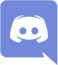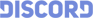#412618Anonymous

I don't have anything on the multiplication table memorized past 5.  You just break down the numbers and square roots to do multiplications past the axis of 5.
For instance, if we wanted 9 x 9.  I would just do 9 x 3(^2).  9 x 3 = 27.  Break down 27 into 20 + 7.  (20+7) x 3.  20 x 3 = 60.  7 x 3 = 21.  60 + 21 = 81.

Which is the samething as 9 x 9 = 81.

Would my life be easier if I memorized everything from 10 x 10?  Yes ~ but someone can say why not memorize 20 x 20 or 200 x 200?  Ditto ~ you learn this sort of math so you can do infinite calculations.  There's more to Math than just memorizing everything like a Monkey.### Slavorum

5 User(s) Online Join Server
•ca\$hbunni
•AphexTwin
•Tujev
•Fia
•Lucifer Morningstar